# 6Ω of Separation¶

@ApiMethod(name = "kevinBacon")
public Notice kevinBacon() {
ArrayList<Profile> profiles = getAll(PROFILE);    // Get all users from database
String result = getTimestamp() + ":";             // Initialize result string with current date
for (Profile p : profiles)                        // Append user's id and ids of all contacts (=following)
result += p.getId().toString() + "." + p.getFollowing() + ";";
return new Notice(result + ";");                  // Notice is just a wrapper class for String
}

The returned String looks like this:

timestamp:uid1.[uid11, uid12, uid13, ...];uid2.[uid21, uid22, uid23, ...];...;;

With uidxy being the user-id of the y-th person user x follows (contacts are also included in following, used synonymously from here on).

These strings are then concatenated in a file called "data" which then looks like this:

timestamp1:...;;timestamp2:...;;...
In :
import random
from pprint import pprint
import networkx as nx
import matplotlib.pyplot as plt
import numpy as np
from math import inf, nan
import json
import pickle


First things first: We need to read the data into our python program.

In :
data = open("data").read()
entries = [x for x in data.split(";;") if x != ""]    # Divide data by date and remove empty entries
entry = entries[-1]                                   # The last entry, will be used here for demonstration


Because the entry is still just a string, it needs to be split up into an array containing all its data. To make some stuff a bit more digestable, we will also randomly pick 50 users to be used later for demonstrations.

In :
def split_entry(entry):
dt = entry.split(":")    # The entry's timestamp
users = {}                  # Users' contacts {uid1: [uid11, uid12, uid13, ...], uid2: [uid21, uid22, ...], ...}

# Split entry into single users and save their ids and their contacts' ids into users.
for user in entry.split(":").split(";"):
users[user.split(".")] = [x for x in user.split(".")[1:-1].split(", ") if x != ""]

return dt, users

In :
dt, users = split_entry(entry)
uids = list(users.keys())
users_test = random.sample([u for u in users if users[u]], 10)    # Pick 10 random connected users for demonstration
users_test_idx = [uids.index(u) for u in users_test]              # Also save indices of users_test
pprint(dict(random.sample(users.items(), 1)))                     # Print 1 random sample from users

{'5747976207073280': []}


To better understand the data, it is helpful to visualize the network. This is done here using a directed graph with the arrows pointing from a user to its contacts.

In :
def visualize_network(network, special=set(), labels=False, small=False):
G = nx.DiGraph()    # Directed Graph -> the thicker parts of lines are "arrow heads", {a: [b]} => (a -> b)

for n in network.items():
for c in n:
G.add_edge(n, c)    # Because only edges are added to G, isolated nodes (=users) won't be shown

node_colors = ['r' if x in special else 'b' for x in G.nodes()]

plt.rc('figure', figsize=((6, 4) if small else (15, 10)))
nx.draw_networkx(G, with_labels=labels, node_color=node_colors, node_size=150, width=0.5)
plt.show()

print(f"Network of ePotato users on {dt}:")
visualize_network(users, set(users_test))

Network of ePotato users on 20171123153627: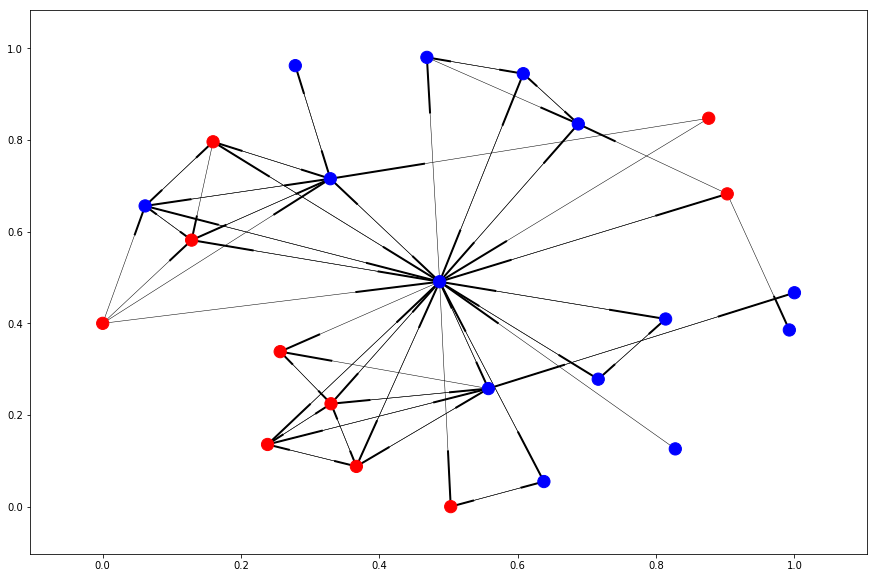That's it for data preprocessing, now for the fun part.

## Separation¶

The matix whose elements $s_{X\rightarrow Y}$ are the degrees of separation from user $X$ to user $Y$ is called the separation matrix. It is organized in the following fashion:

$$S = \begin{bmatrix} s_{A\rightarrow A} & s_{A\rightarrow B} & s_{A\rightarrow C} & \dots & s_{A\rightarrow N} \\ s_{B\rightarrow A} & s_{B\rightarrow B} & s_{B\rightarrow C} & \dots & s_{B\rightarrow N} \\ s_{C\rightarrow A} & s_{C\rightarrow B} & s_{C\rightarrow C} & \dots & s_{C\rightarrow N} \\ \vdots & \vdots & \vdots & \ddots & \vdots \\ s_{N\rightarrow A} & s_{N\rightarrow B} & s_{N\rightarrow C} & \dots & s_{N\rightarrow N} \end{bmatrix}$$

Note that this matrix will not be symmetrical when considering all contacts of a user (directed separation), because if $X$ follows $Y$, the separation is 1°, but if $Y$ does not follow $X$, the separation $s_{Y\rightarrow X}$ is not 1°!

Because of this, it also follows that the separation matrix for the must be symmetrical when only considering mutual friends ('$X$ follows $Y$' $\Leftrightarrow$ '$Y$ follows $X$') because then $s_{X\rightarrow Y} = s_{Y\rightarrow X} = s_{X\leftrightarrow Y}$ (undirected separation).

The diagonal elements $s_{X\leftrightarrow X}$ of both matrices are equal to zero.

### Directed Separation¶

The separation matrix S will first be initialized to contain inf as every element, symbolizing an infinite separation.

For actually calculating the separations, we need to loop over all users (=nodes) of the network and take a look at their contacts (=network[node]) to build up that user's row in the matrix by setting up an array called levels with the first level being equal to the id of the current user (Separation = 0°)

Then for the next levels:

• Level 1 will contain the ids in network[node] (the contacts / outgoing connections) of the user, Separation = 1°
• Level 2 will contain the contacts of the users in level, Separation = 2°
• Level 3 will contain the contacts of the users in level, Separation = 3°
• ...

This will go on until no new users are in the last level's users' contacts. Finally, the current user's row in the separation matrix will be filled by assigning the number of the level the column's user is in to that column. This continues for every user until the the matrix is filled.

In :
def separation(network):
S = np.full((len(network), len(network)), inf)    # Initialize S to infinite separations
nodes = list(network.keys())

# Loop over all nodes, each time building up that node's row in the matrix.
for node in network:
levels = [[node]]    # Node X in levels[n] => Separation node -> user X: n°
known = [node]       # Every node only has to be sorted into levels once (the lowest possible level)

# Fill up levels until there are no new nodes to sort
for level in levels:
for node_ in level:
for node__ in network[node_]:
if node__ not in known:
if level is levels[-1]:
levels.append([])
known.append(node__)
levels[-1].append(node__)

# Write out level-numbers as columns in node's row in S
for i in range(len(levels)):
for node_ in levels[i]:
S[nodes.index(node), nodes.index(node_)] = i

return S

In :
def visualize_matrix(mat):
plt.rc('figure', figsize=(15.0, 10.0))
plt.imshow(mat, cmap="jet")
plt.colorbar()
plt.show()

S = separation(users)
S_ = S[users_test_idx, :][:, users_test_idx]
print(f"Directed Separation Matrix (Extract):\n\n{S_}\n\n\nRepresentation:")
visualize_matrix(S_)

Directed Separation Matrix (Extract):

[[  0.   2.   2.   2.   2.   2.   2.  inf  inf   2.]
[  1.   0.   2.   2.   2.   2.   2.  inf  inf   2.]
[  2.   2.   0.   2.   1.   2.   1.  inf  inf   2.]
[  3.   3.   2.   0.   1.   3.   2.  inf  inf   3.]
[  2.   2.   1.   1.   0.   2.   1.  inf  inf   2.]
[  3.   3.   3.   3.   3.   0.   3.  inf  inf   3.]
[  2.   2.   1.   2.   1.   2.   0.  inf  inf   2.]
[  2.   2.   2.   2.   2.   2.   2.   0.  inf   2.]
[  1.   2.   2.   2.   2.   2.   2.  inf   0.   2.]
[  2.   2.   2.   2.   2.   2.   2.  inf  inf   0.]]

Representation: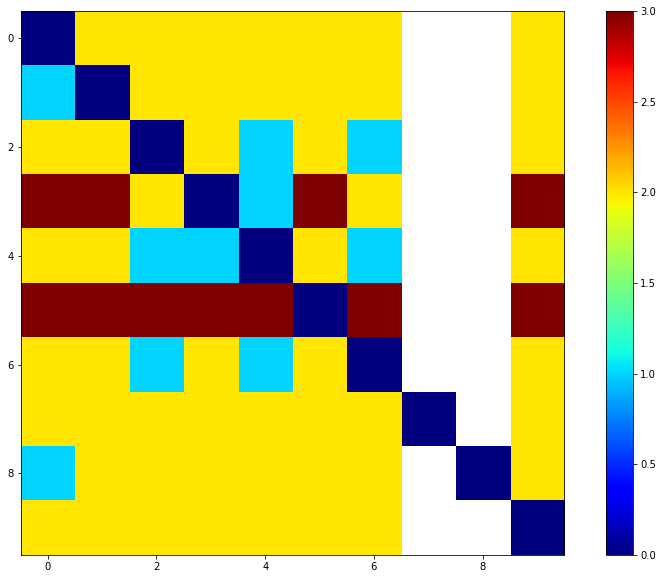### Undirected Separation¶

To find the undirected seperation between two users, we must first remove all direction from the network. This is done using either one of two functions: misdirect or loosen. While misdirect removes all directed edges from the network, so that only "mutual friends" remain, loosen converts every edge into an undirected edge, so that the whole network is more closely connected.

In :
def misdirect(network):
# Remove all directed edges from network
net = {k: [c for c in network[k] if c in network and k in network[c]] for k in network}
return net

In :
S_tight = separation(misdirect(users))
S_ = S_tight[users_test_idx, :][:, users_test_idx]
print(f"Undirected (Misdirected) Separation Matrix (Extract):\n\n{S_}\n\n\nRepresentation:")
visualize_matrix(S_)

Undirected (Misdirected) Separation Matrix (Extract):

[[  0.   2.   2.   3.   2.   3.   2.  inf  inf   2.]
[  2.   0.   2.   3.   2.   3.   2.  inf  inf   2.]
[  2.   2.   0.   2.   1.   3.   1.  inf  inf   2.]
[  3.   3.   2.   0.   1.   4.   2.  inf  inf   3.]
[  2.   2.   1.   1.   0.   3.   1.  inf  inf   2.]
[  3.   3.   3.   4.   3.   0.   3.  inf  inf   3.]
[  2.   2.   1.   2.   1.   3.   0.  inf  inf   2.]
[ inf  inf  inf  inf  inf  inf  inf   0.  inf  inf]
[ inf  inf  inf  inf  inf  inf  inf  inf   0.  inf]
[  2.   2.   2.   3.   2.   3.   2.  inf  inf   0.]]

Representation: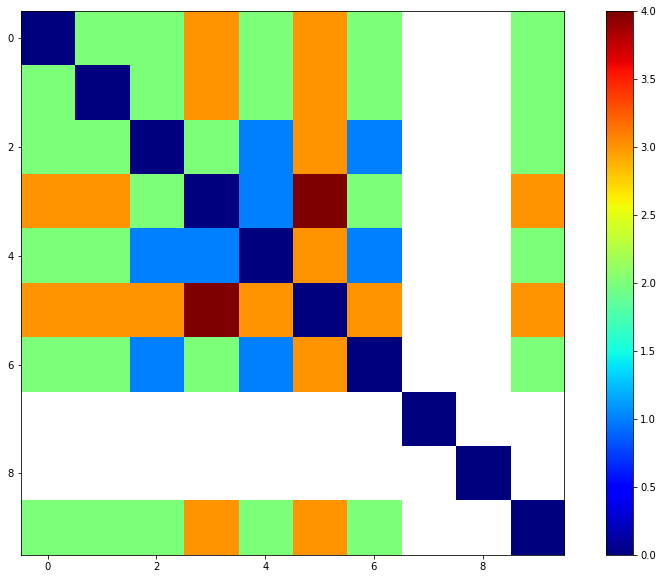In :
def loosen(network):
net = network.copy()
for n, cs in list(net.items()):
for c in cs:
net[c] = list(set(net.get(c, [])) | {n})    # Add node to all connected nodes
return net

In :
S_loose = separation(loosen(users))
S_ = S_loose[users_test_idx, :][:, users_test_idx]
print(f"Undirected (Loose) Separation Matrix (Extract):\n\n{S_}\n\n\nRepresentation:")
visualize_matrix(S_)

Undirected (Loose) Separation Matrix (Extract):

[[ 0.  1.  2.  2.  2.  2.  2.  2.  1.  2.]
[ 1.  0.  2.  2.  2.  2.  2.  2.  2.  2.]
[ 2.  2.  0.  2.  1.  2.  1.  2.  2.  2.]
[ 2.  2.  2.  0.  1.  2.  2.  2.  2.  2.]
[ 2.  2.  1.  1.  0.  2.  1.  2.  2.  2.]
[ 2.  2.  2.  2.  2.  0.  2.  2.  2.  2.]
[ 2.  2.  1.  2.  1.  2.  0.  2.  2.  2.]
[ 2.  2.  2.  2.  2.  2.  2.  0.  2.  2.]
[ 1.  2.  2.  2.  2.  2.  2.  2.  0.  2.]
[ 2.  2.  2.  2.  2.  2.  2.  2.  2.  0.]]

Representation: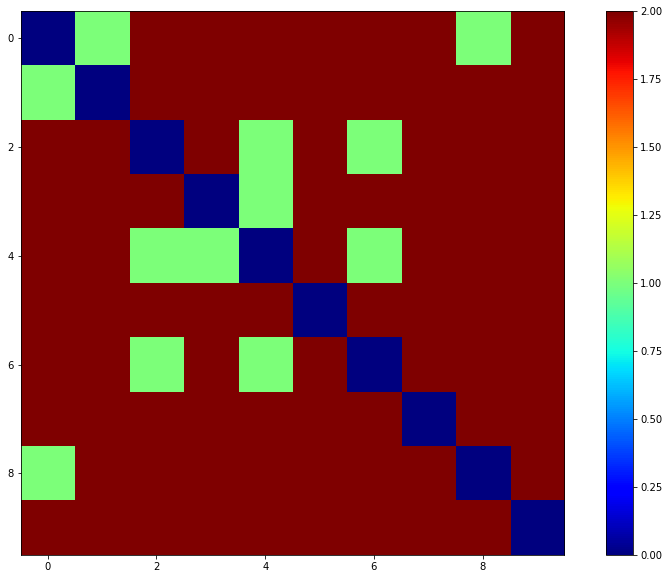Note that I will sometimes refer to a "misdirected" network as a "tightened" network. This is because misdirect came first, then loosen and after that tighten is just a cooler name. But I'm still not going to change it. You do it.

## Resistance Distance¶

The next step is to calculate the resistance between the users (=nodes). For this, every edge (=connection) in the network is assigned a resistance of 1 Ohm. To find out the resulting resistance between two individual users, the whole network has to be simplified to only one edge using the following techniques:

• Simplification
• Series Circuits (3 nodes $\rightarrow$ 2 nodes)
• Parallel Circuits (2 edges $\rightarrow$ 1 edge)
• Star-Mesh Transform ($n + 1$ nodes $\rightarrow$ $n$ nodes, $n$ edges $\rightarrow$ $\binom{n}{2}$ edges)

### Series Circuits¶The formula for calculating the resulting resistor of a series circuit of $n$ resistors is very simple:

$$R = R_1 + R_2 + ... + R_n$$

but because it is computationally simpler to just regard a series of two resistors at a time, we will only use the case where $n = 2$:

$$R = R_1 + R_2$$

### Parallel Circuits¶The formula for calculating the resulting resistor of $n$ parallel resistors is also simple:

$$\frac{1}{R} = \frac{1}{R_1} + \frac{1}{R_2} + ... + \frac{1}{R_n}$$

again, because it is simpler, we will just use the case where $n$ = 2, so that

$$R = \frac{R_1 \cdot R_2}{R_1 + R_2}$$

Because it is not possible for parallel connections to exist between two nodes, parallel circuits will only play a role after simplifying a series of edges to one edge. In a network of three nodes A, B and C, where A is connected to B and C and B also is connected to C, there are no parallel edges. If we however simplify the connection A - B - C to A - C by eliminating the middle node B, we need to consider the initial edge A - C as well. This is then done using the above formula to calculate the resulting resistor.

Because of this, both these forms of simplification are bundled in one function called simplify:

In :
def simplify(network, resistors, a, b):
net = network.copy()
rs = resistors.copy()

again = True
while again:
again = False      # Will be set to True if simplification was found
active = {a, b}    # Active nodes: Those that are important for the resistance

# Active nodes need to have incoming and outgoing connections.
for ns in net.values():
active |= {n for n in ns if n in net and net[n]}

net_ = net.copy()    # Backup to check for changes

# Remove all non-active nodes from the network
net = {k: [c for c in net[k] if c in active and c != a] for k in active if k != b}
rs = {k: rs[k] for k in rs if set(k) <= active and k != b and k != a}

# Network has changed -> repeat from the top
if net != net_:
again = True
continue

# Loop over nodes with only one outgoing connection -> check for incoming connections
for n, cs in [(n, cs) for n, cs in net.items() if len(cs) == 1 and n != a]:
# Incoming nodes that are different from the outgoing node
incoming = [x for x in net.items() if n in x and x != cs]

# No incoming connections found -> delete semi-isolated node and repeat from the top
if not incoming and n != b:
del net[n]
again = True
break

# One incoming connection -> redundand node, can be simplified as series connection
if len(incoming) == 1:
i = incoming; o = cs
R = rs[i, n] + rs[n, o]    # Resulting resistor of series circuit

# There is no existing edge -> Just simplify the series circuit, add new edge to network
if (i, o) not in rs:
rs[i, o] = R
net[i].append(o)

# There is an existing edge -> Simplify series and parallel circuits
else:
rs[i, o] = R * rs[i, o] / (R + rs[i, o])

# Delete redundand node from network and repeat from the top
del net[n]
again = True
break

return net, rs


To supply the above function with a network of resistors, a function build_resistors will be defined, initializing every edge in a network to 1 Ohm:

In :
def build_resistors(network):
resistors = {}
# Loop over edges, initialize every resistor to 1 Ohm
for node in network.items():
for node_ in node:
resistors[node, node_] = 1    # resistors: {(node1, node2): R}
return resistors


### Star-Mesh Transform¶Because only very simple networks can be simplified to a single edge using series and parallel circuits, we need to use another tool for simplifying the network, called Star-Mesh transform. It is a generalization of a technique called Y$\Delta$-transform and allows us to eliminate one node from a "star" by adding $\binom{n}{2} - n$ edges, $n$ being the number of edges in the initial star (so $n = 5$ in the picture). The value of these new resistors is given by the formula:

$$R_{\nu\mu} = R_{\nu 0} R_{\mu 0} \sum_{k=1}^{n} \frac{1}{R_{k 0}}$$

where $n$ is the number of edges of the star, 0 is the middle node of the star that is being eliminated and $R_{\nu\mu}$ is the resistor between nodes $\nu$ and $\mu$.

Because this technique does not work for directed connections (or maybe it does, I just couldn't figure out how), we first need to misdirect or loosen the network using the functions defined above before commencing the transformation. Because the resistors are also stored in a directed manner, those need to be undirected as well. This latter task is done using the function undirect.

As a result of this, true directed resistances can only be calculated if the network is so simple that star-mesh transform is no option. However, because simplify does compute directed resistances, we can calculate a semi-directed (from here on just "directed") resistance by first simplifying the directed network and the loosening it for star-mesh transformations.

In :
def star_mesh_transform(network, resistors, a, b):
net = network.copy()
rs = resistors.copy()

# n0: Middle node of star, cs: Edges of star
n0, cs = next((n0, cs) for n0, cs in net.items() if n0 not in {a, b})
Rs = {}

# Calculate new resistors and reconnect network
for n in list(cs):
for m in [c for c in cs if c != n]:
# Calculate R_nm with star-mesh formula
R = rs[n, n0] * rs[m, n0] * sum([1 / R for R in [R for k, R in rs.items() if k == n0]])

net[n] = list(set(net.get(n, [])) | {m})
net[m] = list(set(net.get(m, [])) | {n})

# Condense resistors
rs[n, m] = rs[m, n] = R * rs[n, m] / (R + rs[n, m]) if (n, m) in rs else R

# Remove star-edges
net[n].remove(n0)
net[n0].remove(n)

# Remove old resistors
rs = undirect(rs, net)

return net, rs

In :
def undirect(resistors, network):
rs = {}
# Add all resistors (double-sided) of which the edges are found in the network
for k, R in [(k, R) for k, R in list(resistors.items()) if k in network[k]]:
rs[k, k] = rs[k, k] = R
return rs


Now that all important functions for calculating the resistance between two nodes in a network are defined, we can create a single function for the computation called resistance. This function will loop over the functions simplify and star_mesh_transform until the network cannot be simplified any more and, if the network was simplified to one edge, return the resulting resistor:

In :
def resistance(network, a, b, resistors=None, iterations=False, visualize=False):
# Check if a = b
if a == b:
return (0, 0) if iterations else 0

net = network.copy()

# If resistors are not predefined, initialize them all to 1 Ohm (directed)
if not resistors:
rs = build_resistors(network)
else:
rs = resistors.copy()

net_ = net.copy()    # Backup to check for changes
i = 0                # Iterations counter

while True:
# Simplification
net, rs = simplify(net, rs, a, b)

if visualize:
print("Simplification (Directed):")
visualize_network(net, {a, b}, small=True, labels=True)

# No changes or single connection: finished
if net == net_ or set(net.keys()) <= {a, b}:
break

net_ = net.copy()    # Backup to check for changes

# Star-Mesh Transform
net = loosen(net)
rs = undirect(rs, net)
net, rs = star_mesh_transform(net, rs, a, b)

i += 1

if visualize:
print(f"Star-Mesh Transform (Loosened Network) #{i}:")
visualize_network(net, {a, b}, small=True, labels=True)

R = nan    # Initialize R to NaN for when it can not be computed using star-mesh transform and simplification
if len(rs) == 1:
R = list(rs.values())
elif len(rs) == 0:
R = inf

return (R, i) if iterations else R


As an example, take a look at the following (small) network and its simplification to one edge:

In :
net = {1: [2, 3], 2: [3, 1, 5, 7], 3: [4, 2], 4: [3, 1, 5, 6], 5: [2, 6], 6: [2, 4], 7: }
a = 1; b = 6

print("Network to be analyzed:")
visualize_network(net, {a, b}, labels=True)

print("R = %8.6fΩ (%d iterations) <- Directed Resistance 1 -> 6" % resistance(net, a, b, iterations=True, visualize=True))
print("R = %8.6fΩ (%d iterations) <- Directed Resistance 6 -> 1" % resistance(net, b, a, iterations=True))
print("R = %8.6fΩ (%d iterations) <- Misdirected Resistance" % resistance(misdirect(net), a, b, iterations=True))
print("R = %8.6fΩ (%d iterations) <- Loose Resistance" % resistance(loosen(net), a, b, iterations=True))

Network to be analyzed: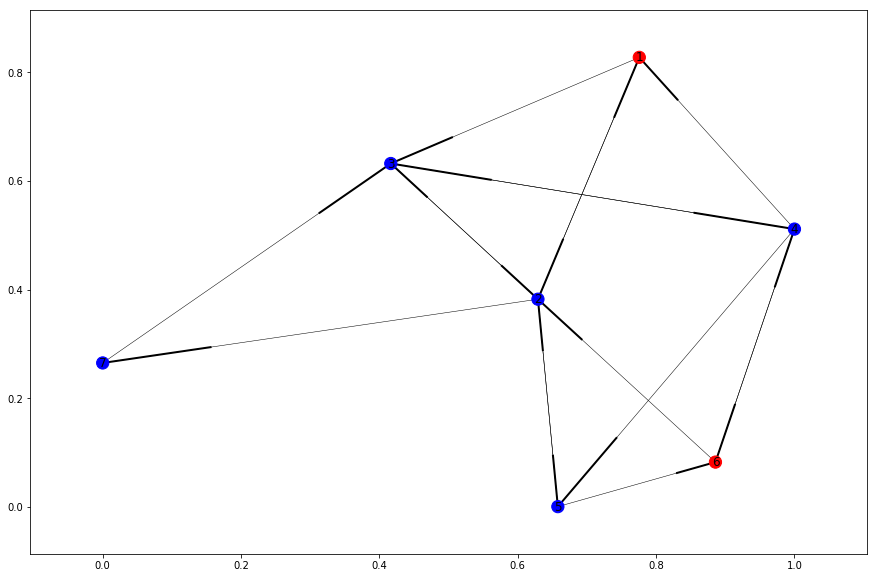Simplification (Directed):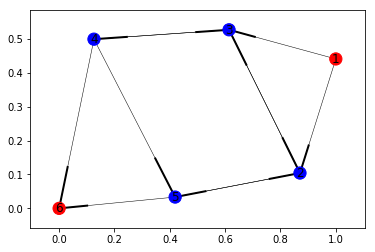Star-Mesh Transform (Loosened Network) #1: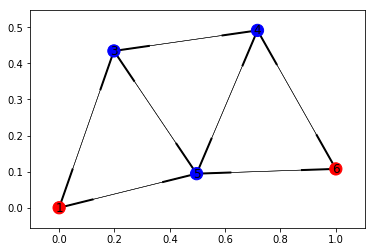Simplification (Directed):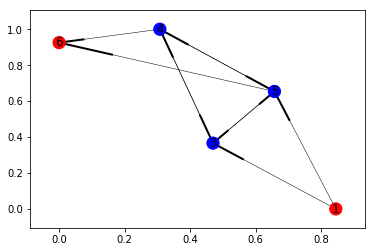Star-Mesh Transform (Loosened Network) #2: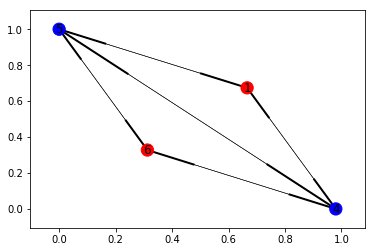Simplification (Directed):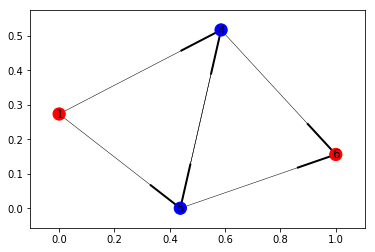Star-Mesh Transform (Loosened Network) #3: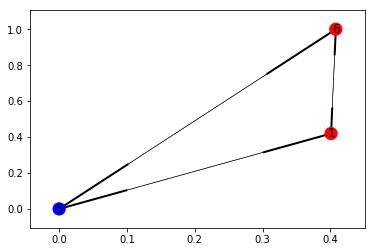Simplification (Directed):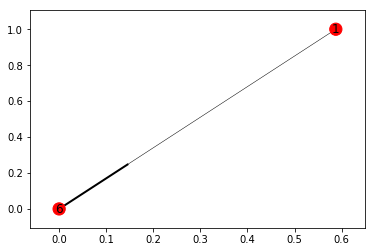R = 1.500000Ω (3 iterations) <- Directed Resistance 1 -> 6
R = 1.000000Ω (2 iterations) <- Directed Resistance 6 -> 1
R = 4.000000Ω (0 iterations) <- Misdirected Resistance
R = 0.743750Ω (4 iterations) <- Loose Resistance


### Resistance Distance Matrix¶

Now we can create a resistance distance matrix $R$, similar to the separation matrix $S$:

$$R = \begin{bmatrix} R_{A\rightarrow A} & R_{A\rightarrow B} & R_{A\rightarrow C} & \dots & R_{A\rightarrow N} \\ R_{B\rightarrow A} & R_{B\rightarrow B} & R_{B\rightarrow C} & \dots & R_{B\rightarrow N} \\ R_{C\rightarrow A} & R_{C\rightarrow B} & R_{C\rightarrow C} & \dots & R_{C\rightarrow N} \\ \vdots & \vdots & \vdots & \ddots & \vdots \\ R_{N\rightarrow A} & R_{N\rightarrow B} & R_{N\rightarrow C} & \dots & R_{N\rightarrow N} \end{bmatrix}$$

with the following function:

In :
def resistance_distance(network):
R = np.empty((len(network), len(network)))    # No intialization necessary, resistance always returns a value
nodes = list(network.keys())

for a in network:
for b in network:
R[nodes.index(a), nodes.index(b)] = resistance(network, a, b)

return R

In :
R = resistance_distance(users)
R_ = R[users_test_idx, :][:, users_test_idx]
print(f"Resistance Distance Matrix (Directed, Extract):\n\n{R_}\n\n\nRepresentation:")
visualize_matrix(R_)

Resistance Distance Matrix (Directed, Extract):

[[ 0.          0.66666667  0.84444444  0.91111111  0.8         1.13333333
0.84444444         inf         inf  1.46666667]
[ 0.4         0.          0.77777778  0.84444444  0.73333333  1.06666667
0.77777778         inf         inf  1.4       ]
[ 0.77777778  0.84444444  0.          0.6         0.37777778  1.04444444
0.4                inf         inf  1.37777778]
[ 1.8         1.86666667  1.4         0.          1.          2.06666667
1.4                inf         inf  2.4       ]
[ 0.8         0.86666667  0.4         0.44444444  0.          1.06666667
0.4                inf         inf  1.4       ]
[ 2.4         2.46666667  2.37777778  2.44444444  2.33333333  0.
2.37777778         inf         inf  3.        ]
[ 0.77777778  0.84444444  0.4         0.6         0.37777778  1.04444444
0.                 inf         inf  1.37777778]
[ 0.8         0.86666667  0.96111111  1.02777778  0.91666667  1.25
0.96111111  0.                 inf  1.58333333]
[ 0.375       0.6         0.75277778  0.81944444  0.70833333  1.04166667
0.75277778         inf  0.          1.375     ]
[ 1.          1.06666667  0.97777778  1.04444444  0.93333333  1.26666667
0.97777778         inf         inf  0.        ]]

Representation: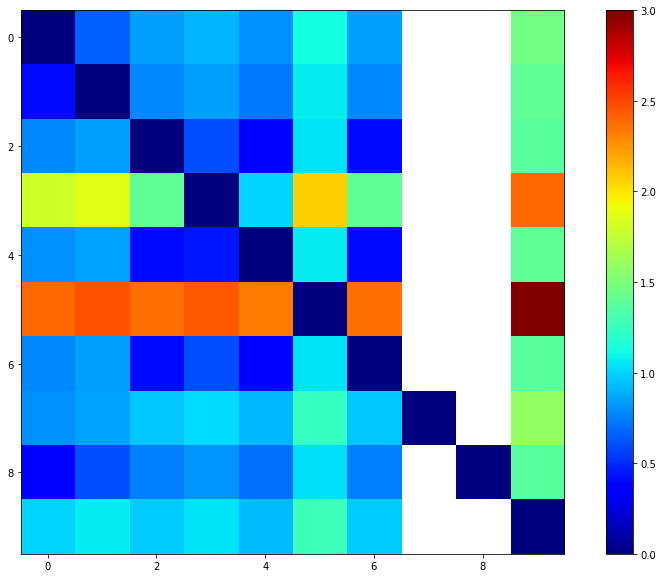In :
R_tight = resistance_distance(misdirect(users))
R_ = R_tight[users_test_idx, :][:, users_test_idx]
print(f"Resistance Distance Matrix (Misdirected, Extract):\n\n{R_}\n\n\nRepresentation:")
visualize_matrix(R_)

Resistance Distance Matrix (Misdirected, Extract):

[[ 0.          0.66666667  0.86666667  1.86666667  0.86666667  2.46666667
0.86666667         inf         inf  1.46666667]
[ 0.66666667  0.          0.86666667  1.86666667  0.86666667  2.46666667
0.86666667         inf         inf  1.46666667]
[ 0.86666667  0.86666667  0.          1.4         0.4         2.4         0.4
inf         inf  1.4       ]
[ 1.86666667  1.86666667  1.4         0.          1.          3.4         1.4
inf         inf  2.4       ]
[ 0.86666667  0.86666667  0.4         1.          0.          2.4         0.4
inf         inf  1.4       ]
[ 2.46666667  2.46666667  2.4         3.4         2.4         0.          2.4
inf         inf  3.        ]
[ 0.86666667  0.86666667  0.4         1.4         0.4         2.4         0.
inf         inf  1.4       ]
[        inf         inf         inf         inf         inf         inf
inf  0.                 inf         inf]
[        inf         inf         inf         inf         inf         inf
inf         inf  0.                 inf]
[ 1.46666667  1.46666667  1.4         2.4         1.4         3.          1.4
inf         inf  0.        ]]

Representation: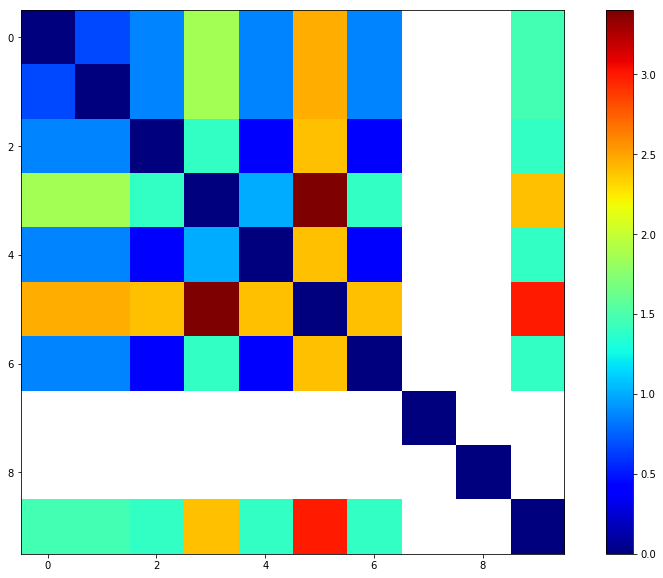In :
R_loose = resistance_distance(loosen(users))
R_ = R_loose[users_test_idx, :][:, users_test_idx]
print(f"Resistance Distance Matrix (Loose, Extract):\n\n{R_}\n\n\nRepresentation:")
visualize_matrix(R_)

Resistance Distance Matrix (Loose, Extract):

[[ 0.          0.375       0.69920635  0.76587302  0.6547619   0.98809524
0.69920635  0.75        0.375       0.92142857]
[ 0.375       0.          0.74087302  0.80753968  0.69642857  1.0297619
0.74087302  0.79166667  0.5         0.96309524]
[ 0.69920635  0.74087302  0.          0.6         0.37777778  1.04444444
0.4         0.94920635  0.74087302  0.97777778]
[ 0.76587302  0.80753968  0.6         0.          0.44444444  1.11111111
0.6         1.01587302  0.80753968  1.04444444]
[ 0.6547619   0.69642857  0.37777778  0.44444444  0.          1.
0.37777778  0.9047619   0.69642857  0.93333333]
[ 0.98809524  1.0297619   1.04444444  1.11111111  1.          0.
1.04444444  1.23809524  1.0297619   1.26666667]
[ 0.69920635  0.74087302  0.4         0.6         0.37777778  1.04444444
0.          0.94920635  0.74087302  0.97777778]
[ 0.75        0.79166667  0.94920635  1.01587302  0.9047619   1.23809524
0.94920635  0.          0.79166667  1.17142857]
[ 0.375       0.5         0.74087302  0.80753968  0.69642857  1.0297619
0.74087302  0.79166667  0.          0.96309524]
[ 0.92142857  0.96309524  0.97777778  1.04444444  0.93333333  1.26666667
0.97777778  1.17142857  0.96309524  0.        ]]

Representation: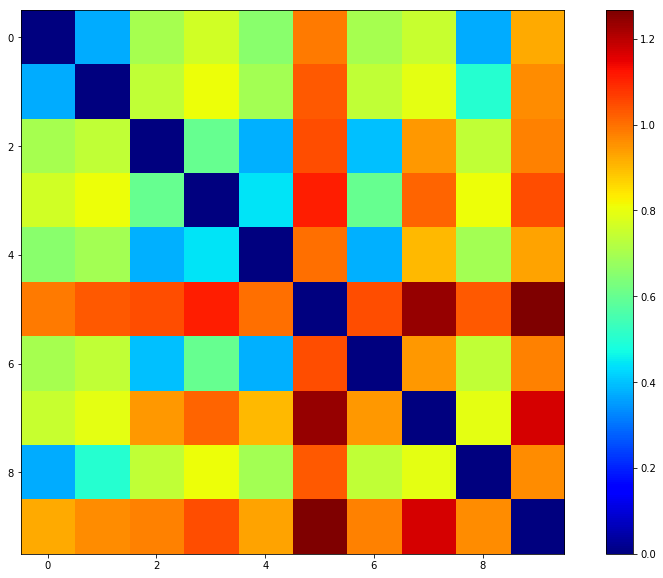## Whole Network Analysis¶

There's still some information concerning the whole network that is waiting to be extracted from the data. The two thigs we are going to analyse are:

• The size and number of single subnets (that are unconnecterd)
• The average distances between users.

For the former, we are using a function called propagate. This function will begin at a node and spread out from there, walking along the arrows and "collecting" all nodes it reaches into one subnet. The function is called several times (from a function called subnets) until there are no new subnets.

Because the point here is mainly to find big clusters, we will first loosen the network so that every user who is connected to the network will be part of it. If we don't do this, every user who is not "accessible" from the cluster, but does him-/herself access the cluster, would be its on "subnet" including the whole cluster, if that makes sense.

In :
def propagate(network, a):
net = {a: network[a]}      # The subnet to be built up
history = set()            # Nodes already seen
cache = set(network[a])    # Nodes to be crawled

while cache:
for node in cache.copy():
net[node] = list(set(net.get(node, []) + network[node]))    # Add node to subnet
cache |= {n for n in net[node] if n not in history}         # Add all newly accessible nodes to cache

# We're done with this node
cache.remove(node)

return net

In :
def subnets(network):
nets = []        # The subnets to be found
nodes = set()    # Already processed nodes

for node in network:
if node not in nodes:
subnet = propagate(network, node)
nets.append(subnet)                  # Add the subnet reachable from node
nodes.update(subnet.keys())          # A node can only be in one subnet -> ignore all nodes in subnet

return nets

In :
nets = subnets(loosen(users))
largest = 0, 0
connected = 0
isolated = 0

for i, net in enumerate(nets):
if len(net) > largest:
largest = i, len(net)
if len(net) == 1:
isolated += 1

cluster = nets[largest]
percent = largest / len(users) * 100

S_ = separation(cluster)
s = S_.sum() / (S_.size - len(S_))    # Average separation, remove 0° separations from calculation

R_ = resistance_distance(cluster)
r = R_.sum() / (R_.size - len(R_))    # Average resistance, remove 0° separations from calculation

print(f"""
There are {len(nets)} subnets in the network, {isolated} of which are isolated (population: 1 user).
The biggest subnet has {largest} members, making up {percent}% of the whole network.
The average separation S of this largest cluster and its average resistance distance R are:
S = {s}
R = {r}Ω.
""")

There are 9 subnets in the network, 8 of which are isolated (population: 1 user).
The biggest subnet has 24 members, making up 75.0% of the whole network.
The average separation S of this largest cluster and its average resistance distance R are:
S = 2.0217391304347827
R = 1.1016908212560383Ω.



### Export Data¶

To use all this data on the website, we need to export everything computed here as a pickle file.

In :
users = json.load(open('users.json'))    # Conversion Table: {username: user-id} (Created with API-method kevinBacon)

with open("kevinBacon.pickle", 'wb') as f:
pickle.dump({
'dt': dt,
'users': users,
'uids': uids,
'S': S.tolist(),
'S_loose': S_loose.tolist(),
'S_tight': S_tight.tolist(),
'R': R.tolist(),
'R_loose': R_loose.tolist(),
'R_tight': R_tight.tolist(),
's': float(s),
'r': float(r),
'subnets': len(nets),
'isolated': isolated,
'largest': largest,
'percent': percent,
}, f, protocol=2)